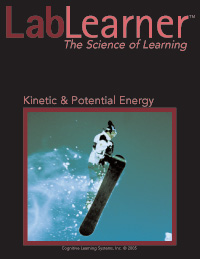# Kinetic & Potential EnergyKinetic & Potential Energy

Through experimentation and data collection, students explore the following type of questions: How does the transfer of potential energy to kinetic energy relate to the Law of Conservation of Energy? Can one form of energy be converted to another? How does converting energy from one form to another relate to the Law of Conservation of Energy?

Students begin this CELL by experimenting with kinetic energy, the energy of motion, and observing the effects of velocity on kinetic energy. Potential energy will be introduced, along with the equation for gravitational potential energy. Students examine the effect of height on potential energy and observe the conversion of potential energy to kinetic energy.

The conversion of potential energy to kinetic energy will be further examined by looking at the conversion of electrical potential energy to kinetic energy in the form of light. Students also observe the transfer of energy from one form of kinetic energy (light) to another form of kinetic energy (thermal energy or heat). Finally, students explore chemical potential energy and its conversion into kinetic energy.

Investigation focus:

• Investigate the Law of Conservation of Energy as it relates to energy transfer and conversion.
• Introduced to two main types of energy: kinetic energy and potential energy.
• Learn that kinetic energy is the energy of motion.
• Study the effects of velocity on kinetic energy
• Demonstrate that energy can be transferred from one object to another.
• Demonstrate that potential energy can be converted into kinetic energy.
• Use the equation for gravitational potential energy: PE = mgh.
• Investigate the conversion of electrical potential energy into light energy and light energy into thermal energy.
• Investigate the conversion of chemical potential energy into kinetic energy.
• Demonstrate mastery of the concepts by determining the potential energy of a sample toy and building two pendulums: one with the same potential energy as the sample and one that produces a higher kinetic energy.